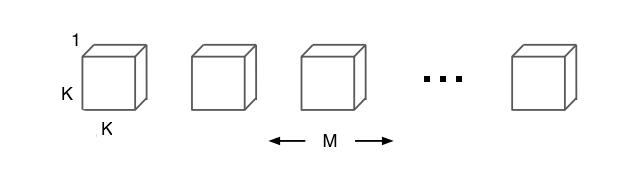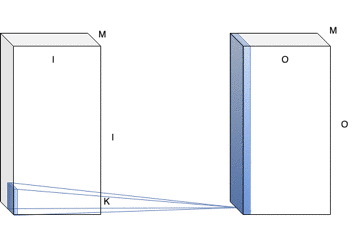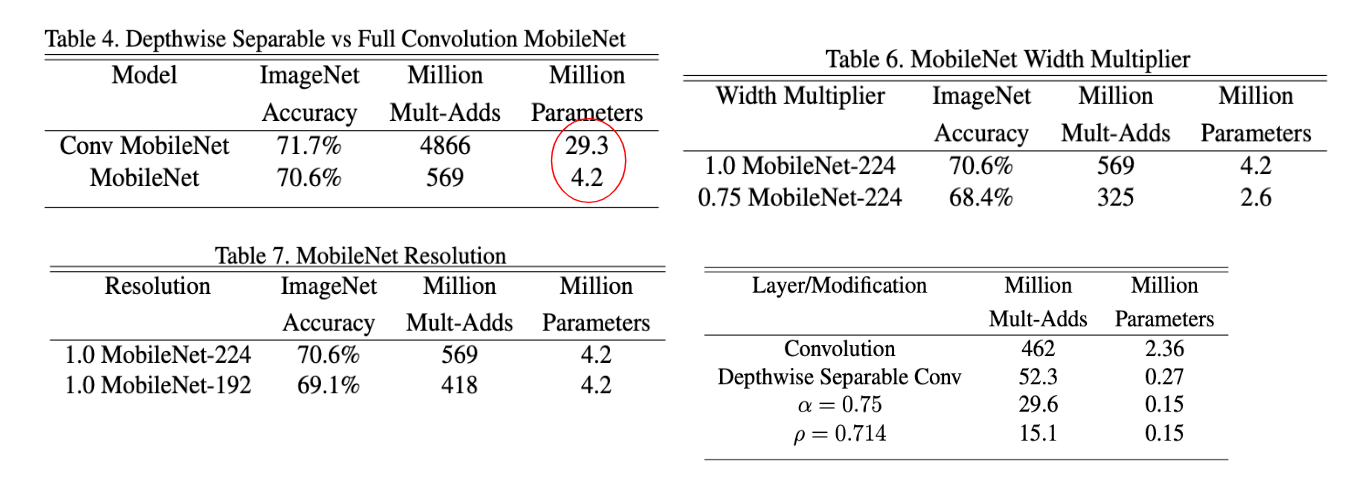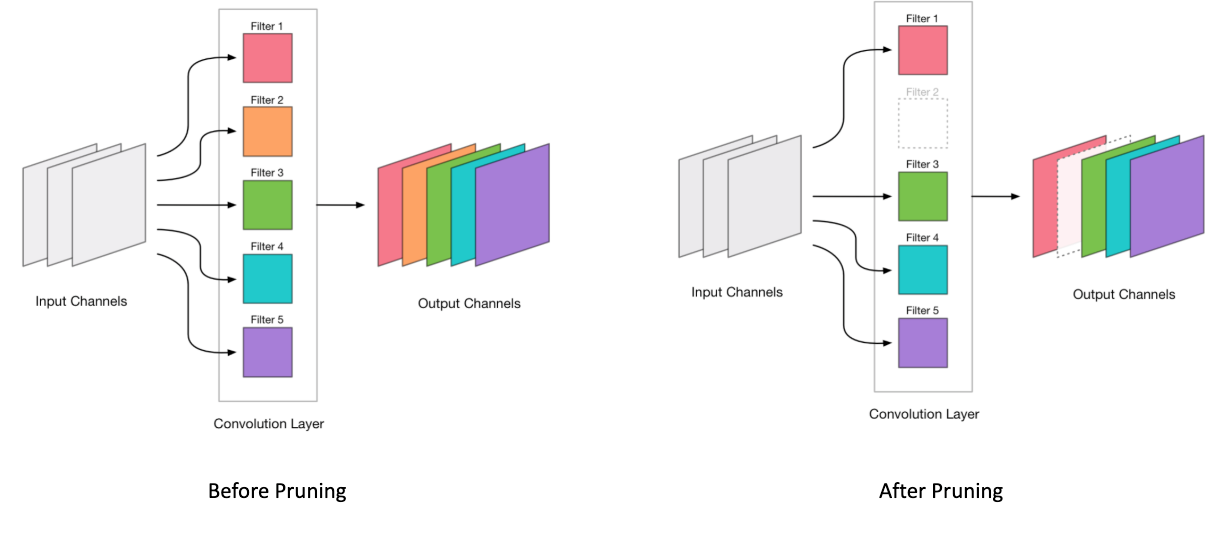# Compression in the AI world: MobileNets, Pruning & Quantisation

## Learn how you can compress a 500 MB large deep learning model to just 5 MB with almost no drop in accuracy.

Over the past couple of years, the state-of-the-art AI algorithms have started moving out from research labs to out in the real world. This has significant implications as the advancements that have been happening in the AI community, now has the potential to reach billions of people across the globe. However, once you decide to deploy an AI model, there are a ton of things that need to be decided, one of them being the nature of the deployment.

There are broadly two ways in which one can deploy an AI model:

While it is easier to deploy your AI model on the server, it has several shortcomings. One of the major ones is the variability of network connection when the model is used on a large scale. Even though the internet seems ubiquitous today, there are still large parts of the world where internet connectivity is intermittent, if not absent. And if your target audience happens to reside in those geographies, you need to consider on-device inference seriously. Some of the other factors that make a case for on-device inference are privacy on the client side and server cost, to name a few.

Now that we understand the need for on-device inference, there are a few significant roadblocks to consider. Firstly, the average size of deep learning models is hundreds of MB; for example, VGGNet is > 500 MB. One cannot deploy such a large model directly on the phone. Second is the inference time. Having a cloud-based model has the benefit that the inference can be done using a GPU, and hence, the inference time can be minimal. That is not the case when you have to run your model on a phone, which has much lower compute power. Finally, one also needs to consider energy efficiency — running your model shouldn’t drain out the battery of the phone completely.

There is an ongoing body of work in the AI research community (including both academia and industry) in the direction of Model Compression — which aims to tackle either all or some of the roadblocks discussed above. In this blog post, I’ll discuss some of the techniques which are commonly used to compress an AI model along with reducing the inference time, while trying to improve energy efficiency. As a guide along this journey, we’ll keep the following metrics in mind while discussing any new technique:

1. Model accuracy drop/rise (rise? yes!)
2. Model size
3. Inference time
4. Energy efficiency

Within model compression itself, there are two broad categories of techniques. One class of compression techniques focus on reducing the model size once the model has been trained. They are referred to as post-training techniques. Pruning and Quantisation are two techniques which belong to this class. The second category, on the other hand, trains a smaller model, to begin with, to match the accuracy of a larger model for the same task. MobileNets (v1 and v2) are an example of this category of techniques.

# MobileNets

We will first discuss the category of techniques which design a smaller and efficient model, to begin with, and train it from scratch. The most widely known work in this class of compression techniques is referred to as MobileNets, which is essentially a toolkit of layers and tricks that make a deep learning model more efficient in terms of runtime while reducing the number of parameters as well. MobileNetv1 discussed the idea of Depthwise Separable Convolution (DS convolution), which is a parameter-efficient way to achieve the output that standard 2D convolution does. It also proposes two hyperparameters — width and resolution multiplier — that can be used to tradeoff between latency and accuracy. I’ll take a stab at explaining the difference between a DS convolution and a regular convolution.

A regular convolution operation takes place as shown below:

Here, the size of the input feature map is `I x I x M` , the kernel (also referred to as filter throughout the post) size is `K x K` and the desired output feature map is of size `O x O x N` . The figure highlights the computation of one cell of one of the channels of the output feature map. To compute one channel of the output feature map requires `K x K x M x I x I` multiplications and additions (referred to as MultAdds from now). For an N-channel output feature map, the total number of MultAdds is, therefore, `K x K x M x I x I x N` .

A standard convolution combines both the filtering step (via the kernel) and the combining of input features (as each filter has a shape of `K x K x M` as shown in the figure above). DS convolution factorises these two operations in two separate steps — depthwise convolution and pointwise convolution — to reduce the total number of parameters as well as the number of MultAdds.

The depthwise convolution step performs only the filtering operation and doesn’t combine the input features. The size of the filters and the depthwise convolution is visually shown below:Filters for the depthwise convolutionNotice how the output feature map size if M and not N (which is the case for the usual convolution).

The Depthwise convolution step is extremely efficient compared to regular convolution as the number of MultAdds involved is only `K x K x M x I x I` .

The pointwise convolution step uses a 1x1 convolution to combine the output of the depthwise convolution to generate the feature map of the desired number of output channels. The number of MultAdds in a pointwise convolution is `M x N x O x O` . Thus, the total number of MultAdds in a Depthwise Separable convolution is: `K x K x M x I x I + M x N x I x I` (I’ve replaced `O x O` by `I x I` in the calculation of the number of MultAdds for pointwise convolution just so that the comparison with a regular convolution is easy and asymptotically, it won’t matter).

Thus, there is a reduction in the number of MultAdds by a factor of 1/K² (using `K x K x M x I x I + M x N x I x I / K x K x M x I x I x N` for the calculation and ignoring the `1/N` term as `N` is usually large). Using a kernel size (K) of 3 gives a reduction by approximately 8–9 times.

The paper also introduced two hyperparameters for tweaking the network architecture to tradeoff between latency and accuracy:
i) Width multiplier (α): The paper refers to the number of channels in each feature map as the width of that feature map. α can be used to make each of the feature maps, and hence, the network, either thinner or thicker. For a DS convolution with the number of input channels `M` and output channels `N`, using a width multiplier, α, makes them `αM` and `αN` respectively. Thus, the number of MultAdds becomes: `K x K x `α`M x I x I + `α`M x `α`N x I x I` . Use α < 1 to reduce the width of the network.
ii) Resolution multiplier (ρ): This is used to reduce the resolution of each of the feature maps in the network. Together with α, the number of MultAdds becomes `K x K x `α`M x `ρ`I x `ρ`I + `α`M x `α`N x `ρ`I x `ρ`I` . This is effectively implemented by reducing the input size.Results of MobileNetv1. The red circle shows the comparison between using a regular convolution vs DS convolution in the MobileNetv1 architecture. As can be seen, there is a large drop in the number of parameters and MultAdds with just a 1% drop in accuracy. Similarly, the other tables indicate how using various hyperparameters affect the relevant metrics.

# Pruning

Now, we shift over to the post-training class of techniques. The first among them is pruning. The broad idea is shown below. You start with the trained model and essentially “cut” or remove some connections such that they don’t have a major effect on the final accuracy. The resulting model is the pruned model which has fewer parameters.

There are a few design choices involved here. One important decision is the criteria based on which certain filters are removed while others are retained. Another crucial factor is the level at which pruning is done. To understand this, consider the figure below.

The figure shows two levels of pruning — one at the level of individual connections between neurons and other at the level of nodes, where individual nodes are removed (and thus, any connection to/from that node).

This design choice is especially relevant for Convolutional Neural Networks, where the connections are non-trivial and instead of removing individual weights within each of the filters, most techniques perform a filter-level pruning, i.e. they either reject or retain an entire filter (which has a shape of `K x K x M` , where the terms refer to their meanings as used in the section above). This is understood better using the image below:After pruning, the filter represented by the dashed line indicates that that filter has been removed. Source: http://machinethink.net/blog/compressing-deep-neural-nets/

Most pruning algorithms actually work in an iterative manner. This means that they prune a few weights, retrain the pruned model to recover from the drop in accuracy as a result of the pruning operation, prune again, and so on. This is done until a threshold on the accuracy drop is reached beyond which the model cannot be pruned further.

As shown above, it is possible to achieve almost no drop in accuracy by pruning almost 95% of the parameters. More details can be found in the original paper here.

# Quantisation

All the weights don’t need to be stored in full-precision, and maybe, even, shouldn’t be stored in full precision. As a crude example, 1.98, 2.11, 2.03 can all be represented simply by 2. The reason why this could be even better than the original model is that it could provide some level of regularization. However, naive approximation might not be the best idea. This paper introduced the idea of trained quantisation, where you can not only perform quantisation in a better way but even train your quantised model further. Also, as shown in the image above, energy efficiency becomes almost thrice when using 16-bit float vs 32-bit float.

The broad idea of trained quantization is to cluster the weight values using K-means clustering where the number of clusters is decided based on the number of bits desired, create a codebook for each weight value, use the codebook to perform a forward pass and get the gradients and update the centroid values of each cluster using the gradient updates. This last step is explained in a bit more detail later.

So, we start with clustering the trained weights and replacing their values with the value of the centroid of the cluster they belong to.

Then, a forward pass is made using the centroid-replaced weight values, and the gradients are calculated accordingly (since each cell goes through a different forward pass, the cells belonging to the same cluster may end up having different gradient values). Since we know which cell indices belong to the same cluster, we group the gradient values based on the cluster the cell indices belong to, and sum over the gradients to receive the gradient value for a cluster. Finally, we perform the usual gradient descent update step on the older centroid values using the gradient value obtained above.

Now, one needs to store only the cluster indices and centroid values. Suppose that 16 clusters are chosen, the weight matrix can be represented using only 4-bits, which is an 8X reduction in model size (asymptotically). Combining this with Huffman coding to represent the bits efficiently, the final model size can be reduced dramatically with only a small drop in accuracy. The combination of Pruning + Quantization + Huffman coding was proposed in the Deep Compression paper which has been referenced throughout this post.

## Resources:

• CS 231N Guest Lecture: Efficient Methods and Hardware for Deep Learning (slides) (video)
• Awesome model compression (Github)
• Official PyTorch implementation for MobileNetv2
• PyTorch implementation of MobileNetv1 compatible for Object Detection
• PyTorch implementation of Pruning (Github)
• PyTorch implementation of Deep Compression (Github)

This post was a very gentle introduction to the world of model compression. There is a ton of work out there that has not been covered, and this is an active area of research in the AI community — with a major push from industry. I intend to write another blog post covering a few more techniques, especially Intrinsic Dimension (from Uber Research) and MobileNetv2 in their gory details. I hope this was a fun read for you and served as a starting point for you to now understand the concepts discussed above in more details as well as become comfortable with the terminologies generally used in this field.

When I was in college, I wanted to be involved in things that would change the world. Now I am.
- Elon Musk

If there’s anything that you might want to share with me or give me any feedback on my writing/thoughts, I would love to hear it from you. Feel free to connect with me on LinkedIn, or follow me on Github. To stay updated with my posts, do follow me on Medium. You can follow our publication on Medium here.